# Length + geometry construction problems - math problems

#### Number of problems found: 14

• Complete constructionConstruct triangle ABC if hypotenuse c = 7 cm and angle ABC = 30 degrees. / Use Thales' theorem - circle /. Measure and write down the length of legs.
• Construct rhombusConstruct rhombus ABCD if given diagonal length | AC | = 8cm, inscribed circle radius r = 1.5cm
• DiagonalsDraw a square ABCD whose diagonals have a length of 6 cm
• Vertex pointsGiven the following points of a triangle: P(-12,6), Q(4,0), R(-8,-6). Graph the triangle. Find the triangle area.
• Two chords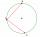There is a given circle k (center S, radius r). From point A which lies on circle k are starting two chords of length r. What angle does chords make? Draw and measure.
• Parallels and one secant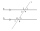There are two different parallel lines a, b and a line c that intersect the two parallel lines. Draw a circle that touches all lines at the same time.
• Outside tangents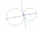Calculate the length of the line segment S1S2 if the circles k1 (S1, 8cm) and k2 (S2,4cm) touch the outside.
• Construct 1Construct a triangle ABC, a = 7 cm, b = 9 cm with right angle at C, construct the axis of all three sides. Measure the length of side c (and write).
• TrianglesIvo wants to draw all the triangles whose two sides of which have a length of 4 cm and 9 cm, and the length of the third side is expressed in whole centimeters. How many triangles does he have?
• Trapezoidal prismCalculate the surface of the quadrilateral prism ABCDA'B'C'D 'with the trapezoidal base ABCD. The height of the prism is 12 cm; ABCD trapezoidal data: AB base length is 8 cm, CD base length is 3 cm, BC arm length is 4 cm, and AC diagonal length is 7 cm. L
• Draw triangle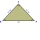Construct an isosceles triangle ABC, if AB = 7cm, the size of the angle ABC is 47°, arms | AC | = | BC |. Measure the size of the BC side in mm.
• Sides od triangleSides of the triangle ABC has length 4 cm, 5 cm and 7 cm. Construct triangle A'B'C' that are similar to triangle ABC which has a circumference of 12 cm.
• Mrak - cloud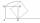It is given segment AB of length 12 cm, where one side of the square MRAK laid on it. MRAK's side length 2 cm shown. MRAK gradually flips along the line segment AB the point R leaves a paper trail. Draw the whole track of point R until square can do the l
• Square gridSquare grid consists of a square with sides of length 1 cm. Draw in it at least three different patterns such that each had a content of 6 cm2 and circumference 12 cm and that their sides is in square grid.

We apologize, but in this category are not a lot of examples.
Do you have an interesting mathematical word problem that you can't solve it? Submit a math problem, and we can try to solve it.

We will send a solution to your e-mail address. Solved examples are also published here. Please enter the e-mail correctly and check whether you don't have a full mailbox.

Please do not submit problems from current active competitions such as Mathematical Olympiad, correspondence seminars etc...

Do you want to convert length units?